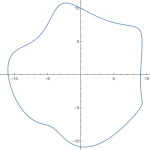# ECE 490: projects for 4th credit

$$\def\Real{\mathbb{R}}$$

1. For a smooth function $$f$$ on $$\Real^n$$, consider the gradient descent algorithm
$x_{n+1}=x_n-\eta\nabla f(x_n)$
(here $$\eta>0$$ is a step size.It is known that for almost all starting positions, the iterations of the gradient descent converge to a local minimizer of $$f$$. To ensure convergence for all initial data, it was suggested to perturb the trajectory once in a while, to escape potential trap in saddles, and shown that the procedure works, at least for non-degenerate critical points.What happens at the degenerate critical points? This project would investigate dependence on the parameters of the algorithm of the time till escape from a vicinity of the origin for
$f(x,y)=x^3+y^3.$
2. Assume that the results of a tournament are given: a table $$T=(t_{kl}), 1\leq k,l\leq N$$, where $$t_{kl}=-t_{lk}$$ is the result of the game(s) of the team $$k$$ against the team $$l$$.
This project will ask, given a predictor function
$\phi(x,y)\to\Real,$
interpreted as the predicted outcome of a match between two teams of strength $$x$$ and $$y$$, respectively, to find the implicit strengths $$x_k, k=1,\ldots,N$$ best fitting observed results $$T$$. In other words, we are looking at minimizing
$\sum_{k,l} L(\phi(x_k,x_l)-t_{k,l})\to\min$
(here $$L$$ is some loss function). One easy example would be to take
$\phi(x,y)=x-y, L(s)=s^2.$
The project is to develop corresponding optimization algorithm and to test it on a real life example (taking as inputs, say, NBA scoreboard).
3. For a polynomial of degree 3 or 4, to determine, the domains where the Newton method for finding its root converges. Namely, we are looking into finding, experimentally, the points $$z_0$$ for which iterations
$z_{n+1}=z_n-\frac{P(z_n)}{P'(z_n)}$
converge (to a root of $$P$$. Write the code and present results for, say, $$P=z^4-5z+1$$.
4. For a closed planar curve $$C$$ bounding a domain, we say that a trajectory $$z_0,z_2,\ldots,z_{p-1}\in C$$ is a $$p$$-periodic billiard trajectory, if for any impact point $$z_k$$, the angle of incidence – the angle of the link $$[z_{k-1},z_k]$$ with the tangent line to $$C$$ at $$z_k$$, – equals the angle of reflection, i.e. the angle of the link $$[z_{k+1},z_k]$$ with the tangent line to $$C$$ at $$z_k$$.It is known that billiard trajectories are the critical points of the length function:$L(z_0,\ldots,z_{p-1})\mapsto \sum_{k=0}^{p-1}|z_k-z_{k+1}|.$
The project will be to search for local maxima of $$L$$ and thus for periodic trajectories for some curves, e.g.
$C=\{(10\cos(t)-\cos(4t),10\sin(t)+\sin(5t-1)), t\in[0,2\pi]\}, p=2,3,7.$
5. Given a function $$f:\Real^d\to\Real$$ and a couple of local minima, $$x_-,x_+$$, how to locate the saddle separating them? One approach is to consider an $$n$$-chain of points, connecting $$x_-$$ and $$x_+$$
$x_-=x_0-x_1-\cdots -x_n=x_+.$
If one minimizes the functional
$\sum_{l=0}^{n-1} f(x_l)+|x_l-x_{l+1}|^2,$
for large $$n$$ the point $$x_l$$ in the chain with the highest $$f(x_l)$$ will be close to the saddle.The project is to develop the code and experiment with it.
6. Consider some collection of lattice vectors, say
$e_1=(1,2), e_2=(-2,1), e_3=(3,2), e_4=(0,2).$
For large $$N$$, there are very many closed trajectories of length $$N$$ with each step being one of the vectors $$e_j$$. These trajectories will contain approximately the same fractions of the vectors of each kind. The project will be to write a code determining these fractions.
4-credit students project signup